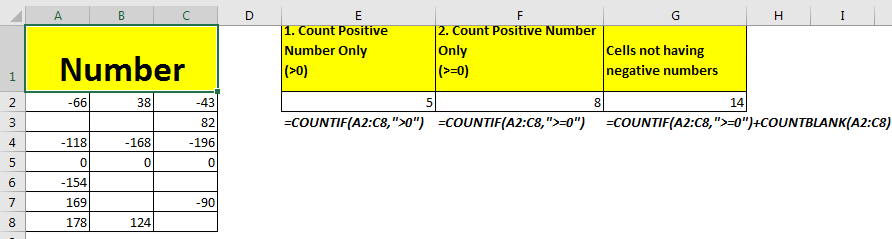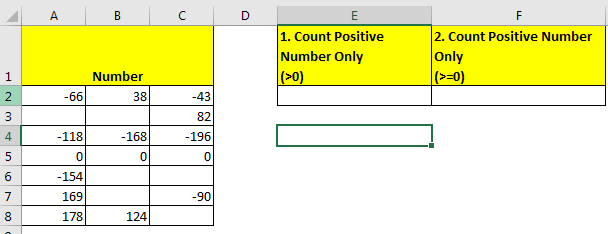# How to Count Positive Values Only in Excel Range in ExcelWhat is a positive number?

I know that you know, but still. Any value/number greater than 0 is a positive number. Numbers equal to 0 or greater than zero are non-negative numbers.

To count cells that contain positive values, only we can use excel COUNTIF function.
The generic formula to Count cell containing positive values only

=COUNTIF(range,”>0”)

Range: the excel range in which you want to count cells having positive numbers (greater than 0).
”>0”: Criteria greater than 0.

The generic formula to count cells containing non-negative numbers.

=COUNTIF(range,”>=0”)

Range: the excel range in which you want to count cells having number non-negative (number greater than or equal to 0).
”>0”: Criteria non-negative values.

Let’s see an example.
Example: Count If Positive Number
Here we have this table that contains some numbers. The table contains some negative numbers, some positive numbers, blank cells, and zeros.
The first task is to count positive numbers only (numbers greater than 0).1. Count if cells containing positive numbers only (>0):
Write this formula in cell E2 to get cell count containing positive numbers.
=COUNTIF(A2:C8,”>0”)

The formula returns 5. Since it contains five positive values that are greater than zero.

2.Count if cells containing non-negative numbers only (>=0):

Write this formula in cell E2 to get cell count containing positive numbers or say non-negative numbers.

=COUNTIF(A2:C8,”>=0”)

The formula returns 8. Because the range includes 0 too. And this formula contains them also.
Note that blank cells are ignored and not counted. To include them too, add the count of blank cells to formula.

=COUNTIF(A2:C8,”>=0”)+COUNTBLANK(A2:C8)

This will return 14.

So this how you can count positive numbers or cells containing positive numbers only. This formula works in all versions of Excel, including excel 2019. Let me know if you have any particular query in regarding this.

Related Articles:

Check If Cell Contains Specific Text

Check A list of Texts In String

COUNTIFS Two Criteria Match

COUNTIFS With OR For Multiple Criteria

Popular Articles

50 Excel Shortcut to Increase Your Productivity: Get faster at your task. These 50 shortcuts will make you work even faster on Excel.

How to use the VLOOKUP Function in Excel: This is one of the most used and popular functions of excel that is used to lookup value from different ranges and sheets.

How to use the COUNTIF function in Excel: Count values with conditions using this amazing function. You don't need to filter your data to count specific values. Countif function is essential to prepare your dashboard.

How to use the SUMIF Function in Excel: This is another dashboard essential function. This helps you sum up values on specific conditions.

Terms and Conditions of use

The applications/code on this site are distributed as is and without warranties or liability. In no event shall the owner of the copyrights, or the authors of the applications/code be liable for any loss of profit, any problems or any damage resulting from the use or evaluation of the applications/code.